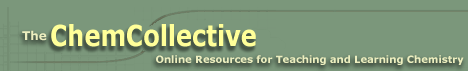The ChemCollective >> CIMS >> Inelastic collisions

# Inelastic collisions

Take a look at the simulation below?. In this simulation, two balls are dropped from the same height. Each ball is composed of the same number of particles. The red and blue particles both have the same mass. The only difference between the two balls is the stiffness of the bonds that join the particles that make up each ball.

You can try running the simulation again by clicking on the button marked "Reset". You can also pause the simulation by clicking on the button labeled "Pause". The bond stiffness of the red and blue bonds can be adjusted using the sliders in the box labeled "Bond Properties". Finally, if you wish to concentrate on the bonds, rather than the atoms, you can use the "Particle Transparency" slider to make the particles mostly transparent or even invisible. This makes it possible to see only the network of bonds that make up each ball.

Here are some experiments you can try:

1. Try setting both the red and blue bond stiffness to the same value. Try this for a few different stiffness values, like 10, 50, and 90. What do you notice about the relationship between the bond stiffness and how high the balls bounce?
2. Does each ball reach the same height as the other? If the balls don't reach the same height on the first and subsequent bounces, what do you think accounts for the difference between them? If the balls were made of 5 x 1023 particles each instead of 72, do you think you would still see the difference?
3. Set the red stiffness to 10 and the blue stiffness to 90 and run the experiment again. Watch closely what happens to the atoms as the ball hits the floor. What is the main difference between the way the two balls deform when they hit the floor? What do you think would happen if the particles weren't bonded to one another?
4. Keep the stiffness settings where they are but make the particles totally transparent. Run the experiment again and watch what happens to the network of bonds between the particles. Do you observe anything you find interesting? What are some words you would use to describe the red network? What about the blue? How do you think those words relate to the stiffness of the bonds in the red and blue networks?